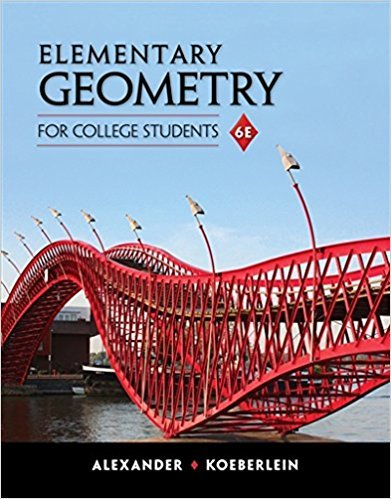×
×

# Given: Right Construct: The median from S toISBN: 9781285195698 295

## Solution for problem 9 Chapter 7

Elementary Geometry for College Students | 6th Edition

• Textbook Solutions
• 2901 Step-by-step solutions solved by professors and subject experts
• Get 24/7 help from StudySoup virtual teaching assistantsElementary Geometry for College Students | 6th Edition

4 5 0 321 Reviews
21
1
Problem 9

Given: Right Construct: The median from S to

Step-by-Step Solution:
Step 1 of 3

.Now that you have the values for x, you can find the values for y by substituting x=2 and x=‐1 into either of the original equations where we solved for y. This will give you: .To check your points of intersection, simply substitute into both of the original equations *From this point on, most graphs will be illustrations from the book Mathematical Models .There will be times when you want...

Step 2 of 3

Step 3 of 3

##### ISBN: 9781285195698

Elementary Geometry for College Students was written by and is associated to the ISBN: 9781285195698. This textbook survival guide was created for the textbook: Elementary Geometry for College Students, edition: 6. The answer to “Given: Right Construct: The median from S to” is broken down into a number of easy to follow steps, and 8 words. This full solution covers the following key subjects: . This expansive textbook survival guide covers 11 chapters, and 543 solutions. The full step-by-step solution to problem: 9 from chapter: 7 was answered by , our top Math solution expert on 01/29/18, 03:43PM. Since the solution to 9 from 7 chapter was answered, more than 214 students have viewed the full step-by-step answer.

Unlock Textbook Solution